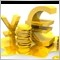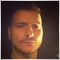# Conditionally selecting which function to run out of 1000s of possibles.11383

Hello everyone.

I am trying to write an EA that has a loop and for each iteration I want it to run a different function

Each function contains some criteria to be checked and its operations are irrelevant to each other so calling function(i) will not do anything.

Naturally I could use a switch function as follows.

```for (int i=0; i<n; i++)
{

switch(i)
{
case '1': function_1(); break;
case '2': function_2(); break;
case '3': function_3(); break;
.
.
.
default:
break;
}

}

void function_1()
void function_2()
.
.
.void function_n()

```

Can anyone recommend a more efficient way to achieve this .

I have seen dynamic indexing is not possible

If possible on each iteration to avoid running the whole switch from the first possible element .

I am thinking something along the lines of referencing using a constant the previous iteration index so the next iteration would not begin looking for the function from all the functions .  Saving the functions in external file if it would provide any benefit.

Any feedback welcome.20106

Michalis Phylactou: Can anyone recommend a more efficient way to achieve this .
No switch, no for.
```function_1();
function_2();
function_3();
⋮```11383

William Roeder:
No switch, no for.

There is some common code to be shared before and after the execution of each function, hence I wanted to perform it like that.17959

Michalis Phylactou:

There is some common code to be shared before and after the execution of each function, hence I wanted to perform it like that.

You dont want a linear execution of all functions from what i understand608

Here is an attempt of calling functions by  indexes.

```#property strict
#property indicator_chart_window
//-----------------------
interface myIntrf{
void fct();
};
//----------------------
class fct1:public myIntrf{
public:
fct1(){};
~fct1(){};
void fct(){Print("my function1");};
};
//-----------------------
class fct2:public myIntrf{
public:
fct2(){};
~fct2(){};
void fct(){Print("my function2");};
};
//------------------------
class fct3:public myIntrf{
public:
fct3(){};
~fct3(){};
void fct(){Print("my function3");};
};
//-----------------------
myIntrf *fctArray;
//+------------------------------------------------------------------+
int OnInit(){
fctArray=new fct1;
fctArray=new fct2;
fctArray=new fct3;
return(INIT_SUCCEEDED);
}
//+------------------------------------------------------------------+
int OnCalculate(const int rates_total,
const int prev_calculated,
const datetime &time[],
const double &open[],
const double &high[],
const double &low[],
const double &close[],
const long &tick_volume[],
const long &volume[],